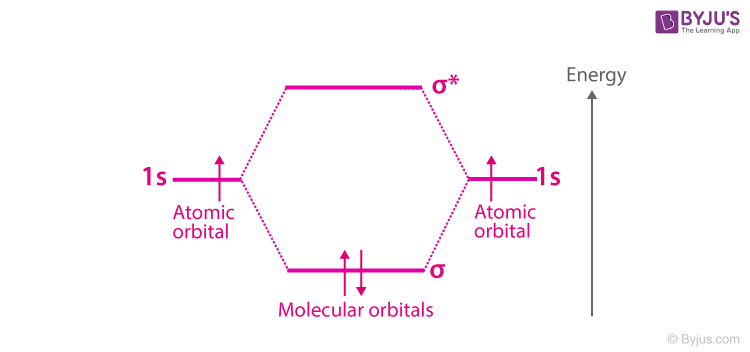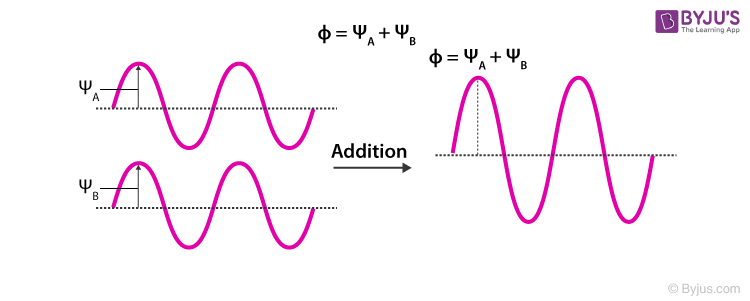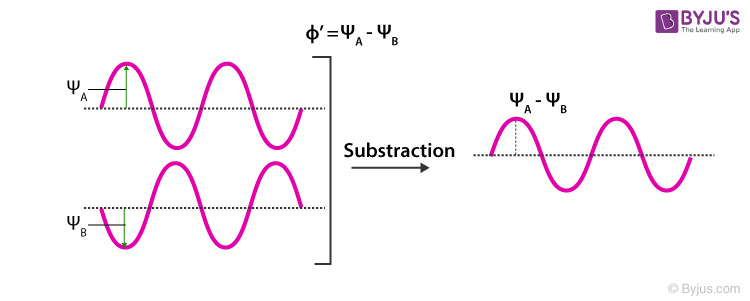Win up to 100% scholarship on Aakash BYJU'S JEE/NEET courses with ABNAT Win up to 100% scholarship on Aakash BYJU'S JEE/NEET courses with ABNAT

# Molecular Orbital Theory

## What Is Molecular Orbital Theory?

The molecular orbital theory (often abbreviated to MOT) is a theory on chemical bonding developed at the beginning of the twentieth century by F. Hund and R. S. Mulliken to describe the structure and properties of different molecules. The valence-bond theory failed to adequately explain how certain molecules contain two or more equivalent bonds whose bond orders lie between that of a single bond and that of a double bond, such as the bonds in resonance-stabilised molecules. This is where the molecular orbital theory proved to be more powerful than the valence-bond theory (since the orbitals described by the MOT reflect the geometries of the molecules to which it is applied).

The key features of the molecular orbital theory are listed below.

• The total number of molecular orbitals formed will always be equal to the total number of atomic orbitals offered by the bonding species.
• There exist different types of molecular orbitals: bonding molecular orbitals, anti-bonding molecular orbitals and non-bonding molecular orbitals. Of these, anti-bonding molecular orbitals will always have higher energy than the parent orbitals, whereas bonding molecular orbitals will always have lower energy than the parent orbitals.
• The electrons are filled into molecular orbitals in the increasing order of orbital energy (from the orbital with the lowest energy to the orbital with the highest energy).
• The most effective combinations of atomic orbitals (for the formation of molecular orbitals) occur when the combining atomic orbitals have similar energies.

In simple terms, the molecular orbital theory states that each atom tends to combine together and form molecular orbitals. As a result of such an arrangement, electrons are found in various atomic orbitals, and they are usually associated with different nuclei. In short, an electron in a molecule can be present anywhere in the molecule.

One of the main impacts of the molecular orbital theory after its formulation is that it paved a new way to understand the process of bonding. With this theory, molecular orbitals are basically considered linear combinations of atomic orbitals. The approximations are further done using the Hartree–Fock (HF) or the density functional theory (DFT) models to the Schrödinger equation.

Molecular orbital theory approximation of the molecular orbitals as linear combinations of atomic orbitals can be illustrated as follows.However, to understand the molecular orbital theory more clearly and in-depth, it is important to understand what atomic and molecular orbitals are first.

## Video Lesson – Molecular Orbital Theory## Linear Combination of Atomic Orbitals (LCAO)

Molecular orbitals can generally be expressed through a linear combination of atomic orbitals (abbreviated to LCAO). These LCAOs are useful in the estimation of the formation of these orbitals in the bonding between the atoms that make up a molecule.

The Schrodinger equation used to describe the electron behaviour for molecular orbitals can be written in a method similar to that for atomic orbitals.

It is an approximate method for representing molecular orbitals. It’s more of a superimposition method where constructive interference of two atomic wave functions produces a bonding molecular orbital, whereas destructive interference produces a non-bonding molecular orbital.

## Conditions for Linear Combination of Atomic Orbitals

The conditions that are required for the linear combination of atomic orbitals are as follows:

### Same Energy of Combining Orbitals

The atomic orbitals combining to form molecular orbitals should have comparable energy. This means that the 2p orbital of an atom can combine with another 2p orbital of another atom, but 1s and 2p cannot combine together, as they have appreciable energy differences.

### Same Symmetry about Molecular Axis

The combining atoms should have the same symmetry around the molecular axis for proper combination; otherwise, the electron density will be sparse. For e.g., all the sub-orbitals of 2p have the same energy, but still, the 2pz orbital of an atom can only combine with a 2pz orbital of another atom but cannot combine with 2px and 2py orbital, as they have a different axis of symmetry. In general, the z-axis is considered the molecular axis of symmetry.

### Proper Overlap between Atomic Orbitals

The two atomic orbitals will combine to form a molecular orbital if the overlap is proper. The greater the extent of overlap of orbitals, the greater will be the nuclear density between the nuclei of the two atoms.

The condition can be understood by two simple requirements. For the formation of a proper molecular orbital, proper energy and orientation are required. For proper energy, the two atomic orbitals should have the same energy, and for the proper orientation, the atomic orbitals should have proper overlap and the same molecular axis of symmetry.

## What Are Molecular Orbitals?

The space in a molecule in which the probability of finding an electron is maximum can be calculated using the molecular orbital function. Molecular orbitals are basically mathematical functions that describe the wave nature of electrons in a given molecule.

These orbitals can be constructed via the combination of hybridized orbitals or atomic orbitals from each atom belonging to the specific molecule. Molecular orbitals provide a great model via the molecular orbital theory to demonstrate the bonding of molecules.

## Types of Molecular Orbitals

According to the molecular orbital theory, there exist three primary types of molecular orbitals that are formed from the linear combination of atomic orbitals. These orbitals are detailed below.

### Anti Bonding Molecular Orbitals

The electron density is concentrated behind the nuclei of the two bonding atoms in anti-bonding molecular orbitals. This results in the nuclei of the two atoms being pulled away from each other. These kinds of orbitals weaken the bond between two atoms.

### Non-Bonding Molecular Orbitals

In the case of non-bonding molecular orbitals, due to a complete lack of symmetry in the compatibility of two bonding atomic orbitals, the molecular orbitals formed have no positive or negative interactions with each other. These types of orbitals do not affect the bond between the two atoms.

## Formation of Molecular Orbitals

An atomic orbital is an electron wave; the waves of the two atomic orbitals may be in phase or out of phase. Suppose ΨA and ΨB represent the amplitude of the electron wave of the atomic orbitals of the two atoms A and B.

Case 1: When the two waves are in phase so that they add up and the amplitude of the wave is Φ= ΨA + ΨBCase 2: When the two waves are out of phase, the waves are subtracted from each other so that the amplitude of the new wave is Φ ´= ΨA – ΨB### Characteristics of Bonding Molecular Orbitals

• The probability of finding the electron in the internuclear region of the bonding molecular orbital is greater than that of combining atomic orbitals.
• The electrons present in the bonding molecular orbital result in the attraction between the two atoms.
• The bonding molecular orbital has lower energy as a result of attraction and, hence, has greater stability than that of the combining atomic orbitals.
• They are formed by the additive effect of the atomic orbitals so that the amplitude of the new wave is given by Φ= ΨA + ΨB
• They are represented by σ, π, and δ.

### Characteristics of Anti-bonding Molecular Orbitals

• The probability of finding the electron in the internuclear region decreases in the anti-bonding molecular orbitals.
• The electrons present in the anti-bonding molecular orbital result in the repulsion between the two atoms.
• The anti-bonding molecular orbitals have higher energy because of the repulsive forces and lower stability.
• They are formed by the subtractive effect of the atomic orbitals. The amplitude of the new wave is given by Φ ´= ΨA – ΨB
• They are represented by σ, π, δ

### Why Are Antibonding Orbitals Higher in Energy?

The energy levels of bonding molecular orbitals are always lower than those of anti-bonding molecular orbitals. This is because the electrons in the orbital are attracted by the nuclei in the case of bonding molecular orbitals, whereas the nuclei repel each other in the case of anti-bonding molecular orbitals.

## Difference between Bonding and Antibonding Molecular Orbitals

 Molecular Orbital Theory Bonding Molecular Orbitals Anti-Bonding Molecular Orbitals Molecular orbitals formed by the additive effect of the atomic orbitals are called bonding molecular orbitals. Molecular orbitals formed by the subtractive effect of atomic are called anti-bonding molecular orbitals. The probability of finding the electrons is more in the case of bonding molecular orbitals. The probability of finding electrons is less in antibonding molecular orbitals. There is also a node between the anti-bonding molecular orbital between two nuclei where the electron density is zero. These are formed by the combination of + and + and – with – part of the electron waves These are formed by the overlap of + with – part. The electron density in the bonding molecular orbital in the internuclear region is high. As a result, the nuclei are shielded from each other and hence the repulsion is very less. The electron density in the antibonding molecular orbital in the internuclear region is very low, so the nuclei are directly exposed to each other. Therefore, the nuclei are less shielded from each other. The bonding molecular orbitals are represented by σ, π, δ. The corresponding anti-bonding molecular orbitals are represented by σ∗ , π∗, δ∗.

The lowering of the energy of the bonding molecular orbital to the combining atomic orbital is called stabilization energy, and similarly, an increase in energy of the anti-bonding molecular orbitals is called destabilization energy.

Try this: Paramagnetic materials, those with unpaired electrons, are attracted by magnetic fields, whereas diamagnetic materials, those with no unpaired electrons, are weakly repelled by such fields. By constructing a molecular orbital picture for each of the following molecules, determine whether it is paramagnetic or diamagnetic.

• B2
• C2
• O2
• NO
• CO

## Features of Molecular Orbital Theory

• The atomic orbitals overlap to form new orbitals called molecular orbitals. When two atomic orbitals overlap, they lose their identity and form new orbitals called molecular orbitals.
• The electrons in the molecules are filled in the new energy states called the molecular orbitals, similar to the electrons in an atom being filled in an energy state called atomic orbitals.
• The probability of finding the electronic distribution in a molecule around its group of nuclei is given by the molecular orbital.
• The two combining atomic orbitals should possess energies of comparable value and similar orientation. For example, 1s can combine with 1s and not with 2s.
• The number of molecular orbitals formed is equal to the number of atomic orbitals combined.
• The shape of molecular orbitals formed depends upon the shape of the combining atomic orbitals.

According to the molecular orbital theory, the filling of orbitals takes place according to the following rules:

Test your knowledge on Molecular Orbital Theory